# Draw Color Filled Shapes in Turtle – Python

## Draw Color Filled Shapes in Turtle – Python

turtle is an inbuilt module in python. It provides drawing using a screen (cardboard) and turtle (pen). To draw something on the screen, we need to move the turtle. To move turtle, there are some functions i.e `forward()``backward()`, etc.

To fill the colors in the shapes drawn by turtle, turtle provides three functions –

fillcolor(): This helps to choose the color for filling the shape. It takes the input parameter as the color name or hex value of the color and fills the upcoming closed geographical objects with the chosen color. Color names are basic color names i.e. red, blue, green, orange.
The hex value of color is a string(starting with ‘#’) of hexadecimal numbers i.e. #RRGGBB. R, G, and B are the hexadecimal numbers (0, 1, 2, 3, 4, 5, 6, 7, 8, 9, A, B, C, D, E, F).

begin_fill(): This function tells turtle that all upcoming closed graphical objects needed to be filled by the chosen color.

end_fill(): this function tells turtle to stop the filling upcoming closed graphical objects.

## Drawing Color Filled Square:

 `# draw color-filled square in turtle` ` ` `import` `turtle` ` ` `# creating turtle pen` `t ``=` `turtle.Turtle()` ` ` `# taking input for the side of the square` `s ``=` `int``(``input``(``"Enter the length of the side of the square: "``))` ` ` `# taking the input for the color` `col ``=` `input``(``"Enter the color name or hex value of color(# RRGGBB): "``)` ` ` `# set the fillcolor` `t.fillcolor(col)` ` ` `# start the filling color` `t.begin_fill()` ` ` `# drawing the square of side s` `for` `_ ``in` `range``(``4``):` `  ``t.forward(s)` `  ``t.right(``90``)` ` ` `# ending the filling of the color` `t.end_fill()`

Input :

```200
green```

Output :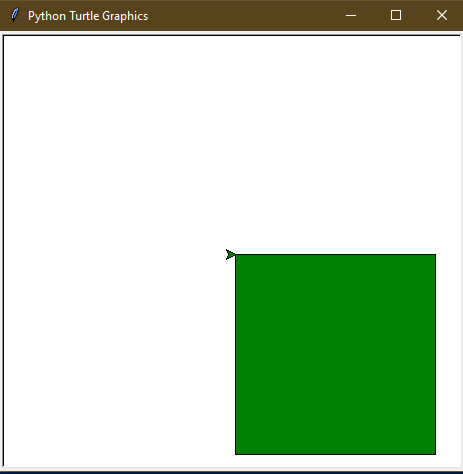## Drawing Color Filled Triangle:

 `# draw color filled triangle in turtle` ` ` `import` `turtle` ` ` `# creating turtle pen` `t ``=` `turtle.Turtle()` ` ` `# taking input for the side of the triangle` `s ``=` `int``(``input``(``"Enter the length of the side of the triangle: "``))` ` ` `# taking the input for the color` `col ``=` `input``(``"Enter the color name or hex value of color(# RRGGBB): "``)` ` ` `# set the fillcolor` `t.fillcolor(col)` ` ` `# start the filling color` `t.begin_fill()` ` ` `# drawing the triangle of side s` `for` `_ ``in` `range``(``3``):` `  ``t.forward(s)` `  ``t.right(``-``120``)` ` ` `# ending the filling of the color` `t.end_fill()`

Input :

```200
red```

Output :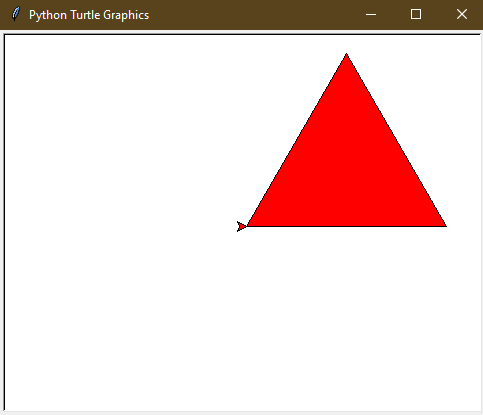## Drawing Color Filled Hexagon:

 `# draw color-filled hexagon in turtle` ` ` `import` `turtle` ` ` `# creating turtle pen` `t ``=` `turtle.Turtle()` ` ` `# taking input for the side of the hexagon` `s ``=` `int``(``input``(``"Enter the length of the side of the hexagon: "``))` ` ` `# taking the input for the color` `col ``=` `input``(``"Enter the color name or hex value of color(# RRGGBB): "``)` ` ` `# set the fillcolor` `t.fillcolor(col)` ` ` `# start the filling color` `t.begin_fill()` ` ` `# drawing the hexagon of side s` `for` `_ ``in` `range``(``6``):` `  ``t.forward(s)` `  ``t.right(``-``60``)` ` ` `# ending the filling of the color` `t.end_fill()`

Input :

```100
#113300```

Output :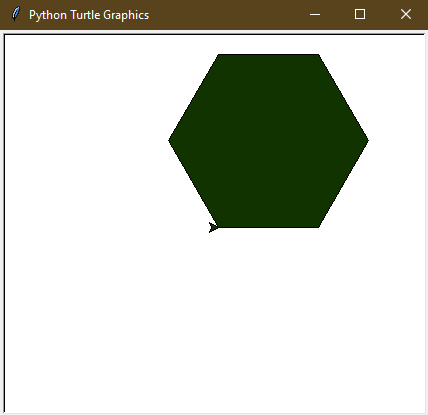## Drawing Color Filled Star:

 `# draw color filled star in turtle` ` ` `import` `turtle` ` ` `# creating turtle pen` `t ``=` `turtle.Turtle()` ` ` `# taking input for the side of the star` `s ``=` `int``(``input``(``"Enter the length of the side of the star: "``))` ` ` `# taking the input for the color` `col ``=` `input``(``"Enter the color name or hex value of color(# RRGGBB): "``)` ` ` `# set the fillcolor` `t.fillcolor(col)` ` ` `# start the filling color` `t.begin_fill()` ` ` `# drawing the star of side s` `for` `_ ``in` `range``(``5``):` `  ``t.forward(s)` `  ``t.right(``144``)` ` ` `# ending the filling of color` `t.end_fill()`

Input :

```200
#551122```

Output :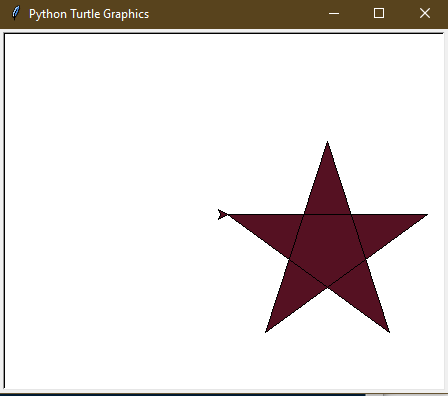## Drawing Color Filled Circle:

 `# draw color filled circle in turtle` ` ` `import` `turtle` ` ` `# creating turtle pen` `t ``=` `turtle.Turtle()` ` ` `# taking input for the radius of the circle` `r ``=` `int``(``input``(``"Enter the radius of the circle: "``))` ` ` `# taking the input for the color` `col ``=` `input``(``"Enter the color name or hex value of color(# RRGGBB): "``)` ` ` `# set the fillcolor` `t.fillcolor(col)` ` ` `# start the filling color` `t.begin_fill()` ` ` `# drawing the circle of radius r` `t.circle(r)` ` ` `# ending the filling of the color` `t.end_fill()`

Input :

```100
blue```

Output :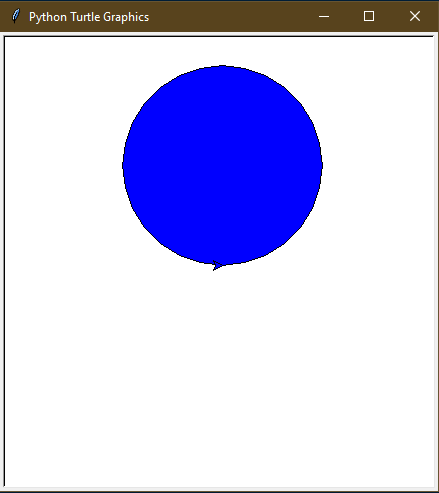Last Updated on November 13, 2021 by admin

## Authentication using Python requestsAuthentication using Python requests

Authentication using Python requests Authentication refers to giving a user permissions to access a particular

## Python – How to sort a list of stringsPython – How to sort a list of strings

Python | How to sort a list of strings Given a list of strings, the

## Dunder or magic methods in PythonDunder or magic methods in Python

Dunder or magic methods in Python Dunder or magic methods in Python are the methods having two

## How to input multiple values from user in one line in Python?How to input multiple values from user in one line in Python?

How to input multiple values from user in one line in Python? For instance, in

## Python – Call Parent class methodPython – Call Parent class method

Python: Call Parent class method A class is a user-defined blueprint or prototype from which

## Python DecoratorsPython Decorators

Decorators in Python Decorators are a very powerful and useful tool in Python since it allows

## Reading and Writing to text files in PythonReading and Writing to text files in Python

Python provides inbuilt functions for creating, writing and reading files. There are two types of

## How to add Empty Column to Dataframe in Pandas?How to add Empty Column to Dataframe in Pandas?

Add Empty Column to Dataframe in Pandas In data Frames, Empty columns are defined and